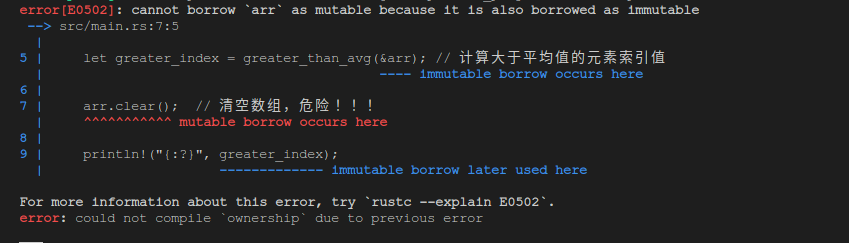•# 理解Rust中的所有权概念

## 所有权是什么 ?

1. 使用一个垃圾回收器持续不断的寻找那些不再使用的内存，例如 Java 语言。
2. 程序员必须手动分配和释放内存，例如, C++ 语言。

1. 通过带有一系列规则的一个所有权系统来管理内存，编译器会在编译期间检测代码是否遵循了这些规则。

### 关于栈和堆

• 在很多编程语言中，并不需要经常思考关于栈（Stack）和堆（Heap）的问题，但是对于像 Rust 这样的系统编程语言，一个值是在栈上还是堆上对于语言的行为以及为什么我们会作出某些决定有很大影响。
• 栈和堆都是我们的代码在运行过程中可以使用的内存部分，但是他们组织数据的方式不同。
• 栈的数据结构类似于一垒盘子，存数据时只能在最顶部添加，移除数据时也只能从最顶部移除（Last In First Out)，而且存取的数据必须是已知固定大小的 —— 已知是指在编译期间已知。
• 堆的数据结构不像栈那样紧凑，比较松散，用于存储在编译期间还不知道大小或者大小有可能会改变的数据。当我们要将数据存储到堆上时，系统会在堆上找一个还未占用且足够大的空间，然后将其标记为已经占用，然后返回给我们一个指向那块空间的指针，这个过程被称为 —— 分配（Allocating）, 因为指针的大小是固定的，所以可以将指针存储在栈上。
• 由于栈上的数据紧凑且大小固定，而访问堆上的数据需要根据指针的指向寻找数据 —— 需要频繁在相距很远的数据之间跳转，所以访问堆上数据的速度比访问栈上数据的速度更慢。
• 当我们调用一个函数时，传入函数的值（包括指向堆上数据的指针）都会被推入（Push）栈中，当函数结束，这些值会被弹出（Pop off）栈。
• 持续跟踪哪些代码正在使用堆上的哪些数据，以最小化堆上的重复数据并清理不使用的数据，以防止耗尽空间正是所有权要解决的问题。

### 所有权规则

1. Rust 中的每个值都有一个变量 —— 该变量的所有者（owner）
2. 在一个时间段内只能有一个所有者
3. 当所有者超出作用域范围，值会被删除

### 变量的作用域

``````fn main() { // 在这里 s 是无效的，因为它还没有被声明
let s = "hello"; // s 从这里开始生效
// 接下来可以开始使用 s 做些事情
}//s 的作用域从这里结束，s不再有效

``````

1. s 出现在作用域中，它开始有效
2. 一直保持有效，直到该作用域结束

### String 类型

#### 字面量（Literal）

``````let s = "hello";
``````

``````let mut s = String::from("你好");
s.push_str(",小明");

println!("s: {}", s);
``````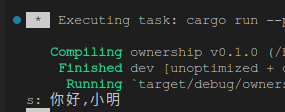### 内存和分配

• 由于字面量是固定值——无法修改，被硬编码到源代码中，最终被编译到可执行程序中，当程序运行时会被加载到内存中。所以在编译时就已经确定占用的内存空间——无法存储大小不确定的内容。
• 而标准库中提供的 String 类型则不同 —— 它是将数据存储到堆上，所以可以存储在编译时还无法确定大小的内容，而且可以被修改。

1. 在运行时向内存分配器申请空间
2. 当超出作用域范围时，要将申请到的分配空间还回去

``````let mut s = String::from("你好");
``````

1. 通过垃圾回收器GC(garbage collector)，持续跟踪不再被需要的内存并回收， 像Java使用这种方式。这种方式的优缺点是:
- 优点是程序员不需要关注内存回收的问题，不像rust有权限规则的限制
- 缺点是由于使用了GC导致更复杂的运行时环境，然后运行性能较慢
2. 程序员手动回收，需要程序员去判断哪个变量不再被需要，然后手动释放内存，像 C/C++ 使用这种方式，这种方式的优缺点是：
- 优点是运行时环境简单，所以运行性能好
- 缺点是需要程序员手动释放变量的内存空间，而且每个空间的申请和释放必须是一对一匹配的，如果释放的太早会造成变量过早无效，如果忘记释放又会造成内存泄漏，如果重复释放也会造成程序崩溃，所以程序员编写代码繁琐而且容易出错。

1. 一旦变量超出作用域的范围，其内存空间会被自动回收
``````{
let s = String::from("hello"); // s 变量从现在开始生效
// 接下来可以操作变量 s
}//该作用域结束，s变量不再有效

``````

#### 通过(移动)Move实现变量和数据的交互

##### 标量数据类型
``````   let mut x = 5;
let y = x;
println!("x: {}, y: {}", x, y);

x = 6;
println!("x: {}, y: {}", x, y);
``````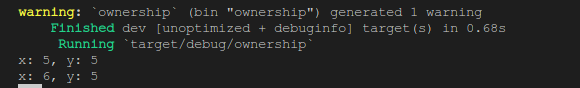##### 复杂类型
``````let x = String::from("hello");
let y = x;
``````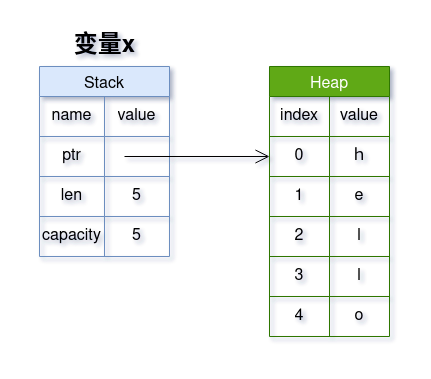1. 栈（Stack）中，存放元数据:
- ptr: 指针，指向存放字符串的堆内存地址
- len: 字符串占用的字节长度
- capacity: 变量x向内存分配器申请到的堆内存大小，单位也是字节
2. 堆（Heap）中，存放字符串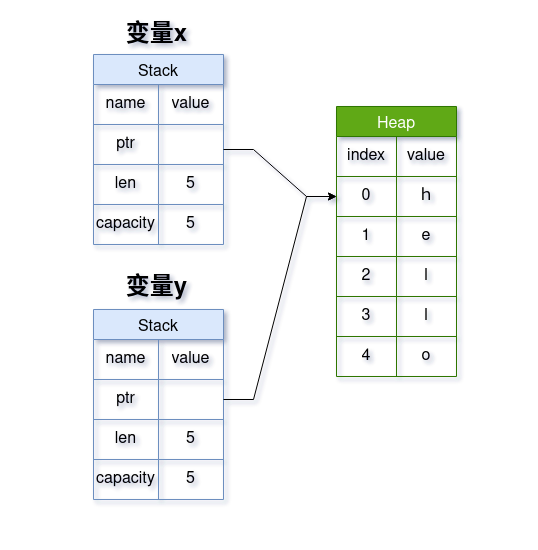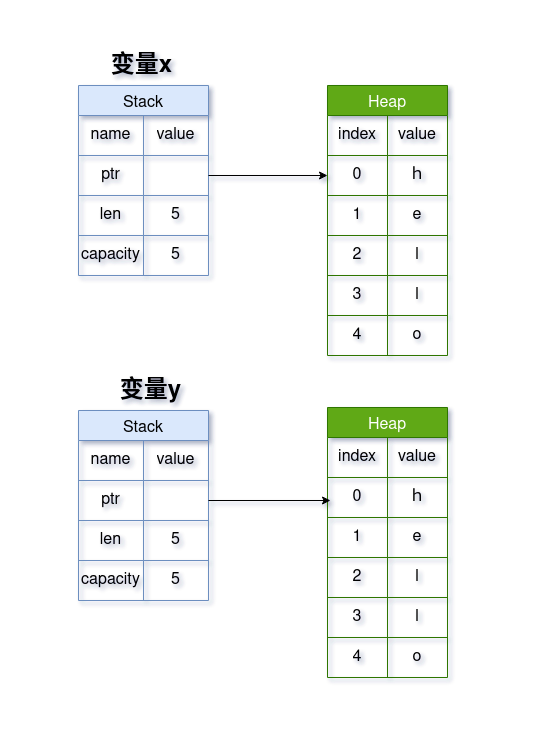``````let x = String::from("hello");
let y = x;
println!("x: {}", x);
``````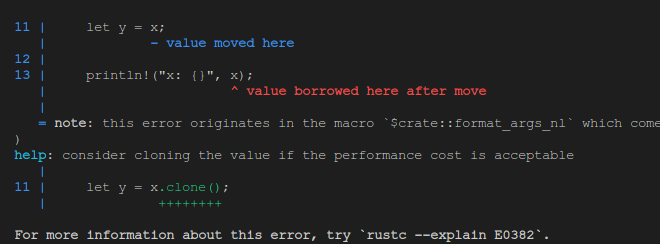#### 通过克隆（Clone）实现变量与数据的交互

##### 复杂类型

`````` let x = String::from("hello");
let y = x.clone();
println!("x: {}, y: {}", x, y);
``````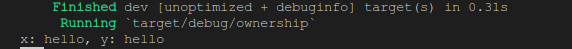##### Copy trait

Rust中的 `trait`类似于 java 中的接口 `interface` 、抽象`abstract`方法或`default`方法等，实现某个`trait`的类型需要实现这个 `trait`中指定的一些方法，所以实现这个`trait`的类型会有一些共通的特性。

• 这个类型或者该类型包含的子元素的类型没有实现`Drop`trait 。

Rust中实现`Copy`trait的数据类型有:

1. 所有的整数类型
2. 所有的布尔类型
3. 所有的浮点类型
4. 所有的字符类型
5. 所有子元素类型都实现了`Copy`trait的tuple

### 所有权和函数

#### 传递函数参数和作用域

``````fn main() { // 在这里 s,x 是无效的，因为它们还没有被声明

let s = String::from("hello"); // 复杂类型 String 变量 s 开始生效，进入作用域

takes_ownership(s); // 变量 s 赋予函数参数变量 some_string, 变量 s 失效
// 变量 s 失效，不可以再被使用
// println!("调用take_ownership函数后的s: {}", s); //发生编译错误

let x = 5; // 整型变量 x 开始生效，进入作用域

makes_copy(x); // 变量 x 赋予函数参数变量 some_integer, 因为整型实现了 Copy trait, 所以直接复制

// 变量 x 依然有效，可以继续使用
println!("调用make_copy函数后的x: {}", x);

} // 变量 x、 s 超出作用域，变量 x 被从栈中弹出，变量 s 的所有权已经被转给函数变量 some_string 所以不需要释放s的内存

fn takes_ownership(some_string: String) {// 函数参数变量 some_string开始生效，进入作用域
println!("some_string: {}", some_string);
} // 函数参数变量 some_string　超出作用域，Rust调用 drop 方法释放它的内存

fn makes_copy(some_integer: i32) { // 函数参数变量 some_integer 开始生效，进入作用域
println!("some_integer: {}", some_integer);
} // 函数参数变量 some_integer 超出作用域，被弹出栈
``````

#### 返回值和作用域

``````fn main() { // 在这里 s1,s2,s3 是无效的，因为它们还没有被声明

let s1 = gives_ownership(); // gives_ownership 将其返回值赋予了变量 s1, 因为返回类型是 String, 所以将权限Move到了s1
// s1开始生效，进入作用域

let s2 = String::from("hello"); // 复杂类型 String 变量 s2 开始生效，进入作用域

let s3 = takes_and_gives_back(s2); // 因为s2是String类型，将所有权Move到了函数参数变量a_string,
// 变量s2失效，无法再被使用，函数takes_and_gives_back将返回值所有权
//赋予了变量 s3, 变量s3开始生效，进入作用域

} // 变量 s3,s2,s1 超出作用域，由于s2已经失效，Rust调用 drop 方法释放 s1,s3的内存

fn gives_ownership() -> String {
let some_string = String::from("yours"); // 变量 some_string生效，进入作用域
some_string // 变量 some_string 将所有权返回给调用函数，some_string变量失效
} // some_string 变量无效，无需调用drop方法释放内存

fn takes_and_gives_back(a_string: String) -> String { // 变量 a_string 生效并获得所有权，进入作用域
a_string // 变量 a_string 将所有权返回给调用函数，a_string 失效
}// a_string 变量已经无效，无需调用 drop 方法释放内存
``````

``````fn main() {
let s1 = String::from("hello");
let (s2, len) = calculate_length(s1);

println!("'{}' 的长度是： {}", s2, len);
}

fn calculate_length(s: String) -> (String, usize) {
let length = s.len();
(s, length)
}
``````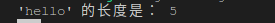## 引用和借用（Borrowing）

### 引用的借用

``````fn main() {
let s1 = String::from("hello");

let len = calculate_length(&s1);

println!("'{}'的长度是: {}", s1, len);

}

fn calculate_length(s: &String) -> usize {
s.len()
}
``````

1. 调用函数calculate_length函数时传入的是`&s1` 而不是 `s1`
2. calculate_length 函数的参数类型是 `&String` 而不是 `String`
3. calculate_length 函数的返回类型是 `usize` 而不是 `(String, usize)`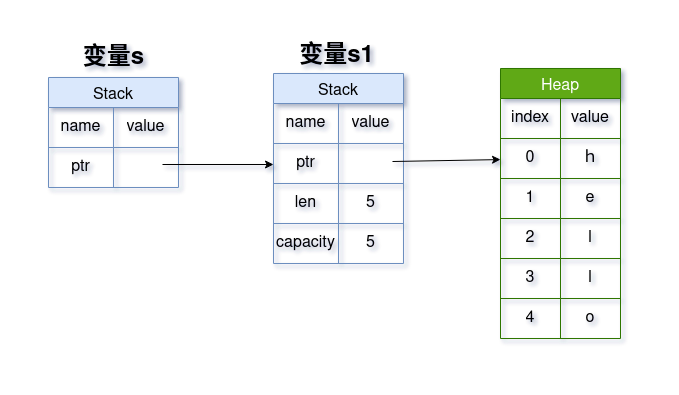### 引用的修改

``````fn main() {
let s1 = String::from("hello");
change(&s1);
}

fn change(s: &String) {
s.push_str(", world");
}
``````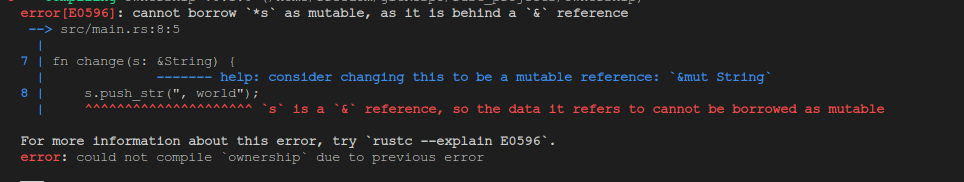Rust中的引用和变量一样，默认是以不可修改的方式借用，如果要以可修改的方式借用也是在变量前加 `mut`关键字

``````fn main() {
let mut s1 = String::from("hello");
change(&mut s1);

println!("修改后的s1: {}", s1);
}

fn change(s: &mut String) {
s.push_str(", world");
}
``````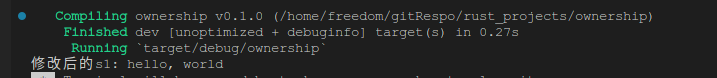why ? 其实这个也很好理解，如果变量是不可修改变量，那就意味这它的数据内容是不可修改的，毕竟变量 `s1`才是数据的所有者，如果所有者都无法修改，那么我们这个借用者 `&mut s1` 又有什么资格去修改呢，所以借用者`&mut s1`可以修改的前提是 —— 所有者指定其数据内容是可以被修改的。

1. 再有可修改引用
2. 再有不可修改引用

#### 再有可修改引用

``````fn main() {
let mut s1 = String::from("hello");

let r1 = &mut s1;
let r2 = &mut s1;

println!("r1: {}, r2: {}", r1, r2);
}
``````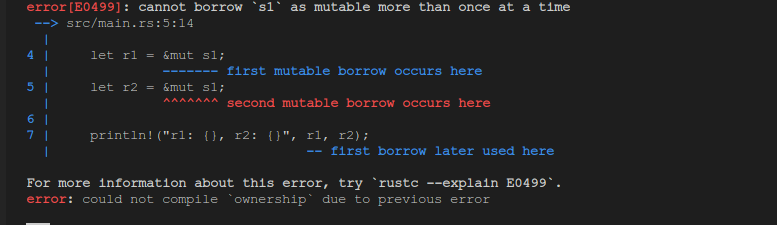``````fn main() {
let mut s1 = String::from("hello");

let r1 = &mut s1;

println!("r1: {}", r1);

let r2 = &mut s1;

println!("r2: {}", r2);
}
``````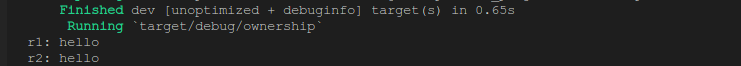why ? 为什么我们只是将第二个可修改引用的创建放到 println 后边就没问题了呢？

``````fn main() {
let mut s1 = String::from("hello");
{
let r1 = &mut s1;
}
let r2 = &mut s1;

println!("r2: {}", r2);
}
``````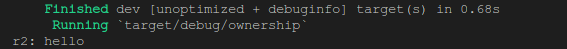#### 再有不可修改引用

``````fn main() {
let mut s1 = String::from("hello");

let r2 = &mut s1;
let r3 = &s1;

println!("r2: {}, r3: {}", r2, r3);
}
``````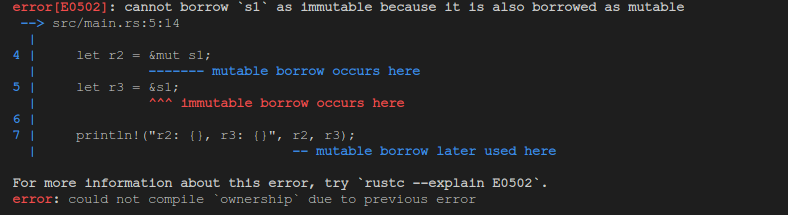``````fn main() {
let mut s1 = String::from("hello");

let r2 = &mut s1;

println!("r2: {}", r2);

let r3 = &s1;

println!("r3: {}", r3);
}
``````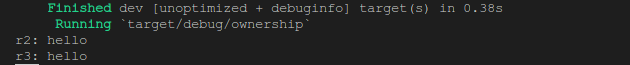``````fn main() {
let mut s1 = String::from("hello");

let r2 = &mut s1;

println!("r2: 不打印");

let r3 = &s1;

println!("r3: {}", r3);
}
``````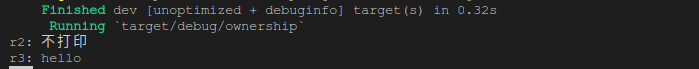``````fn main() {
let mut s1 = String::from("hello");

let r2 = &mut s1;
let r3 = &s1;

println!("r3: {}", r3);
}
``````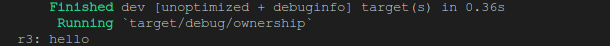`不可修改引用的生命周期`不可以与`同一个变量的其他引用（&和&mut）的生命周期`发生`重叠`

``````fn main() {
let mut s1 = String::from("hello");

let r2 = &mut s1;

s1 = String::from(", World");

println!("r2: {}", r2);

}
``````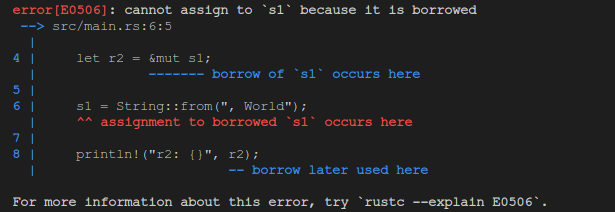``````fn main() {
let mut s1 = String::from("hello");

let r2 = &s1;

s1 = String::from(", World");

println!("r2: {}", r2);

}
``````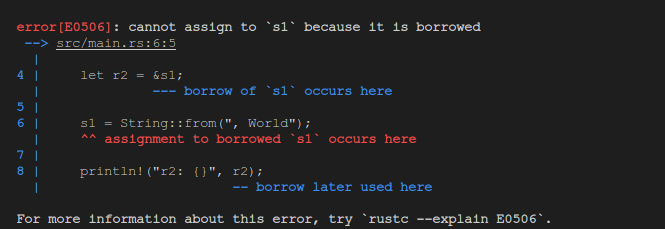`可修改引用`的生命周期期间，不可以引入对该变量的其他引用（无论是&还是&mut）而且数据内容不可以通过其变量修改；同样的在`不可修改引用`的生命周期期间，不可以引入可修改引用或通过变量修改数据内容。

#### 再借用(Reborrowing)

``````fn main() {
let mut s1 = String::from("hello");

let r2 = &mut s1;

s1.push_str(", world");

println!("r2: {}", r2);

}
``````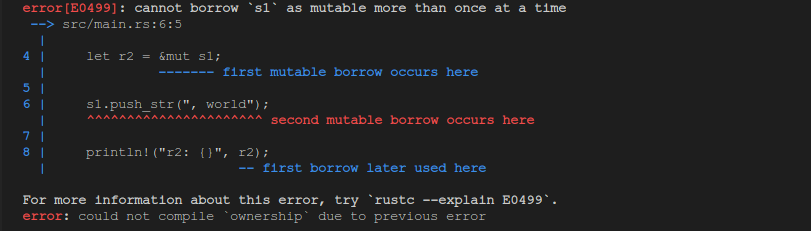``````fn main() {
let mut s1 = String::from("hello");

let r2 = &mut s1;

r2.push_str(", world");

println!("r2: {}", r2);

}
``````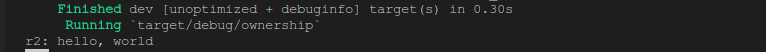// TODO: 关于再借用的内容先留空，后边再解决…

### 悬挂引用（Dangling）

``````fn main() {

let dangling_reference = dangle();

}

fn dangle() -> &String {

let s1 = String::from("hello"); // 变量 s1 生效，进入作用域范围

&s1 // 返回一个对刚才创建的字符串的引用
} // 变量 s1 超出作用域范围，调用 drop 方法，字符串内存被释放，引用&s1指向了无效的内存
``````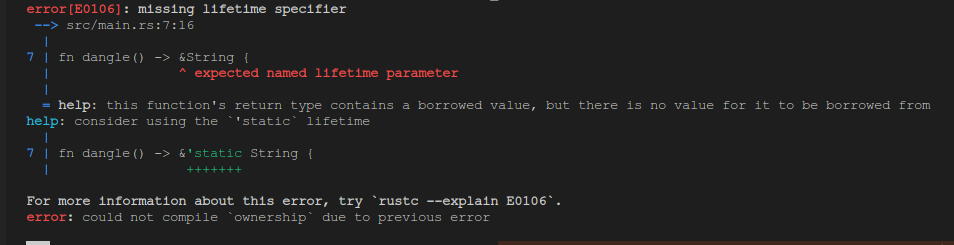## 切片类型（Slice）

Rust 中的切片类型也是一种引用，不过它不像我们上边提到的引用直接引用的是变量在内存中的整块数据内容，而是引用其整块内容的一个切片。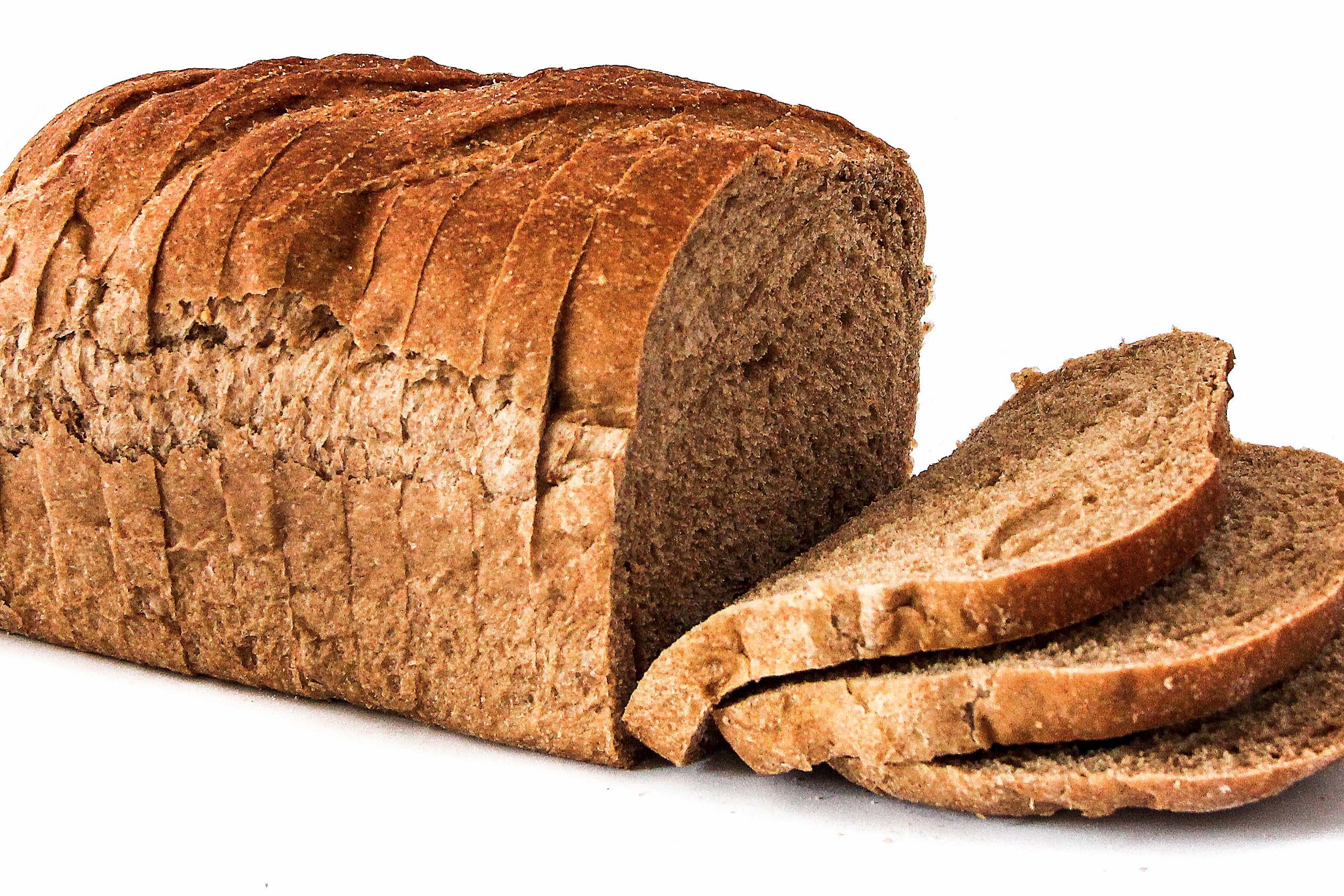### 字符串切片

``````fn main() {

let s1 = String::from("hello world");

let hello: &str = &s1[0..5];  // 通过指定开始索引和结束索引确定切片的范围
let world = &s1[6..11]; // 切片内容包含开始索引，不包含结束索引
let world1 = &s1[6..s1.len()]; // 索引可以使用变量

println!("hello: {}, world: {}, world1: {}", hello, world, world1);

let hello2 = &s1[..5]; // 如果开始索引从0开始，可以省略
let world2 = &s1[6..]; // 如果结束索引到结尾，可以省略
let whole = &s1[..];   // 如果开始索引从0开始，结束索引到结尾，都可以省略

println!("hello2: {}, world2: {}, whole: {}", hello2, world2, whole);

}
``````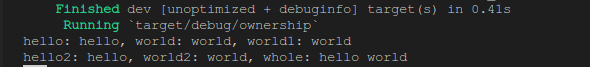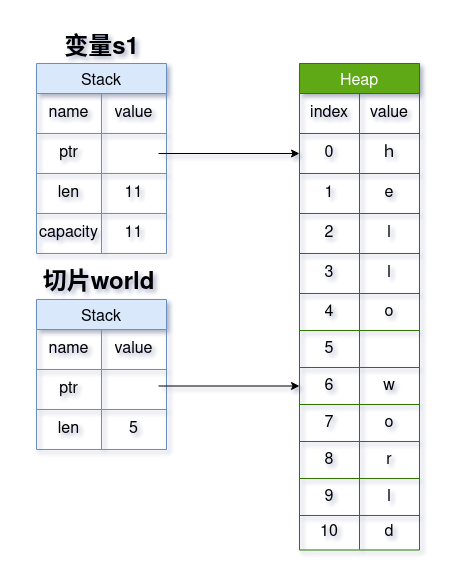### 数组切片

``````fn main() {

let arr = [1, 2, 3, 4, 5, 6];

let slice1 = &arr[0..5];
let slice2 = &arr[5..6];

println!("slice1:{:?}, slice2: {:?}", slice1, slice2);

let slice11 = &arr[..5];
let slice22 = &arr[5..];
let whole = &arr[..];

println!("slice11: {:?}, slice22: {:?}, whole: {:?}", slice11, slice22, whole);

}
``````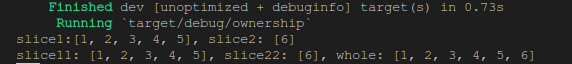### 切片的优势

``````fn main() {

let mut arr = vec![1.3, 4.5, 8.3, 10.5];

let greater_index = greater_than_avg(&arr); // 计算大于平均值的元素索引值

arr.clear();  // 清空数组，危险！！！

println!("{}", greater_index);

}

fn greater_than_avg(arr: &[f32]) -> usize {

let sum: f32 = arr.iter().sum(); // 计算所有元素的总和

let avg = sum / arr.len() as f32; // 计算平均值

for (index, item) in arr.iter().enumerate() {

if item > &avg{ // 如果大于平均值
return index; // 返回索引值
}

}

arr.len() // 只有一个元素，或者为空的情况
}
``````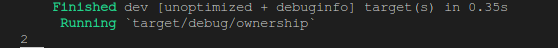``````fn main() {

let mut arr = vec![1.3, 4.5, 8.3, 10.5];

let greater_index = greater_than_avg(&arr); // 计算大于平均值的元素索引值

arr.clear();  // 清空数组，危险！！！

println!("{:?}", greater_index);

}

fn greater_than_avg(arr: &[f32]) -> &[f32] {

let sum: f32 = arr.iter().sum(); // 计算所有元素的总和

let avg = sum / arr.len() as f32; // 计算平均值

for (index, item) in arr.iter().enumerate() {

if item > &avg{ // 如果大于平均值
return &arr[index..]; // 返回切片
}

}

&arr[arr.len()..] // 只有一个元素，或者为空的情况
}
``````